# Multiplication

Multiplication
Go back to  'PEMDAS'

## Introduction to Multiplication

Multiplication is one of the four basic arithmetic operations. Operations that can be applied to different math concepts like multiplying and dividing fractions, decimals, rationals, integers etc. These operations form the building blocks for the other math concepts.

You are at the grocery store, and you buy $$5$$ bags of chips each costing $$\text{Rs }15/-.$$ But before you get to the checkout line, you want to figure out the total and hand in the right amount of change to the cashier. How do you calculate? $$15 + 15 + 15 + 15 + 15 = 75$$

Multiplication might seem as a tough task at first, but when you break it down and practice the basics, you will have it figured out in no time. All multiplication is, is repeated addition.

## Multiplication using objects.

Multiplication at lower grades is best introduced as repeated addition. In grades $$1$$ and $$2$$ it is the first time that kids are getting to know what multiplication is. To begin with multiplication should be introduced using objects. That’s actually all there is to it. Simple right? The image given below shows 4 groups of 3 apples each which is nothing but 4 x 3 = 12.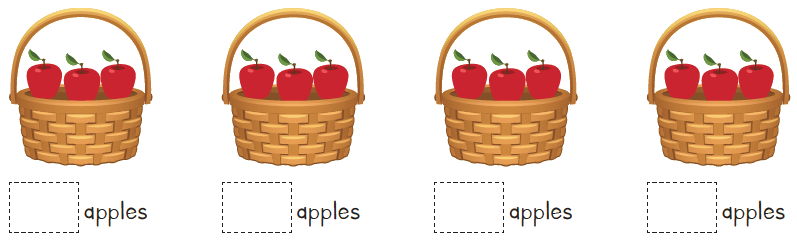There are $$3$$ apples in each basket.

In all there are $$12$$ apples.

$$3 + 3 + 3 + 3 = 12$$

This can also be written as  $$4 \text{ times } 3 =12$$

Which can be written as  $$4 \times 3 = 12$$

\begin{align}& \text{Number of Baskets} \qquad \qquad \text{Number of Apples per Basket} \qquad \qquad \text{(Total Number of Apples)}\\\\ & \text{(Groups)} \qquad \qquad \qquad \qquad \qquad \;\; \text{(Items per Group)} \qquad \qquad \qquad \qquad \qquad \;\; \text{(Items)}\end{align}

Here's another one.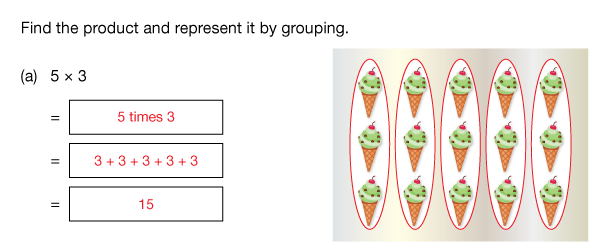## Topics closely related to Multiplication and Division

It is important, however, that, before multiplication is taught formally, children should be familiar with the following experiences and skills.

Children should:

1. Be able to count securely

2. Understand basic addition and subtraction

3. Be able to form groupings of the same size

Comfort with multiplying and dividing quantities can help in high school math and also math in our everyday lives.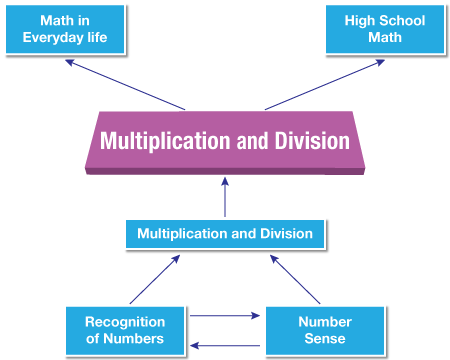## Multiplication using dots

After the idea has been introduced using objects, dot arrays can be used to strengthen this idea.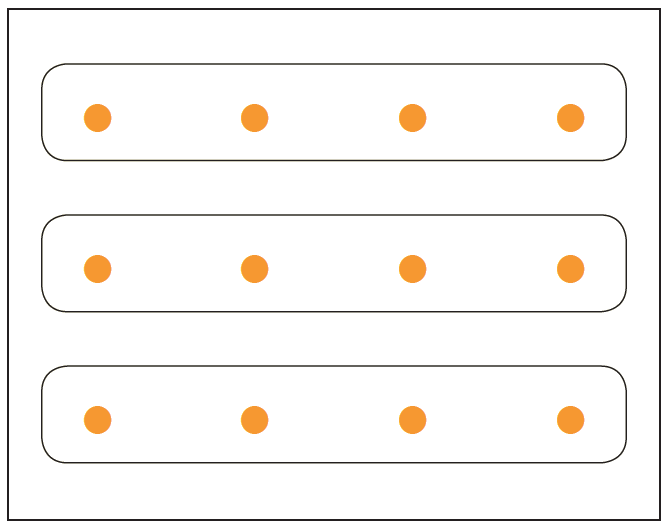$$4 + 4 + 4 = 12$$## Multiplication using number line

Eventually when the children are comfortable with the idea of multiplication using objects, it is time to introduce it on a number line. This time using only the numbers.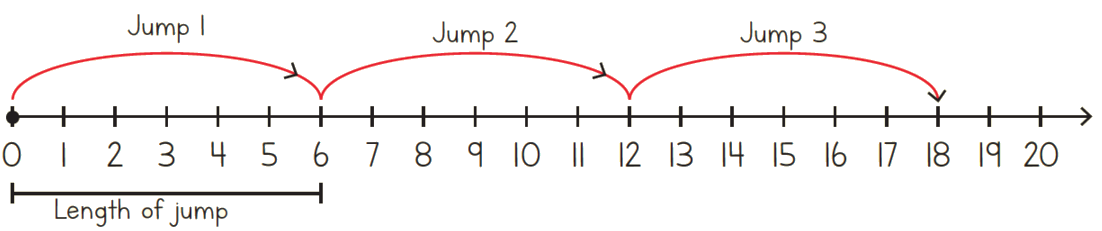The representation can also be written as $$6 + 6 + 6 = 18.$$ The multiplication statement can be written as,## Building multiplication tables

Multiplication tables can be built using the dot arrays. Understanding this would make the retention of tables easier. Given below is an example of building tables using dot arrays,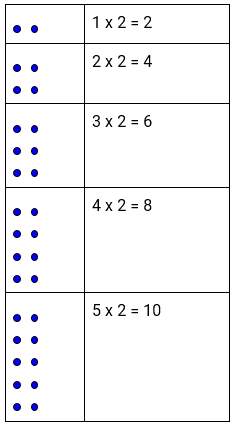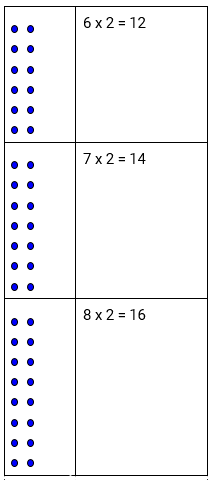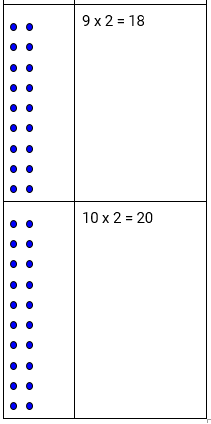Another way to build multiplication tables is by using a 100 grid.

 X 0 1 2 3 4 5 6 7 8 9 10 1 0 1 2 3 4 5 6 7 8 9 10 2 0 2 4 6 8 10 12 14 16 18 20 3 4 5 6 7 8 9 10

## Flow of multiplication

Given below is the progression that should be adopted while teaching multiplication.

1. Moving from unitary counting to counting in multiples;

2. The notion of repeated addition (including number line modelling) and doubling;

3. Introduction of multiplication symbol.

4. Learning multiplication facts;

5. Simple scaling (by whole number scale factors) in the context of measurement or money;

6. Multiplication using standard algorithms ;

## Procedure - Multiplication using standard algorithm

Let’s try and understanding how do we multiply numbers using standard algorithm.

Example 1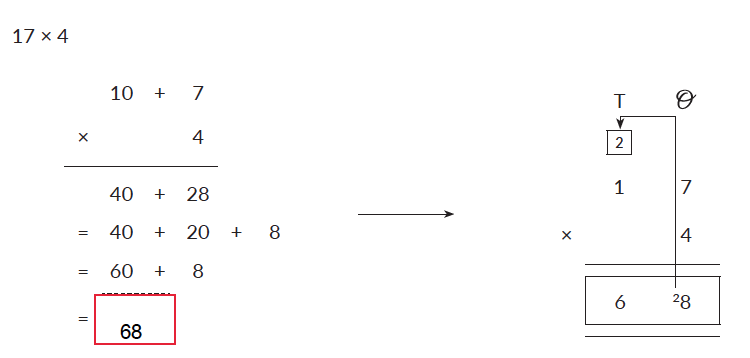In the given example $$17 \times 4$$ is nothing but $$4 (10 + 7).$$ As you can see $$4$$ is multiplied by $$7$$ first and then $$10$$ giving an answer of $$40 + 28.$$ $$28$$ can also be written as $$20 + 8.$$ Figure 2 explains how $$2$$ is carried over to the tens place add there are $$2$$ tens. After adding the $$2$$ tens to $$4$$ tens the answer obtained is $$6$$ tens. The final product is $$6 \text{ tens and } 8 \text{ ones.}$$

Example 2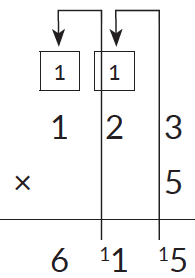In this example as you can see $$5$$ multiplied by $$3$$ is $$15.$$ $$15$$ is $$1 \text{ ten and } 5 \text{ ones.}$$ The ones are written under the ones place. The remaining $$1 \text{ ten }$$ is carried over to the tens place. This is how the carryover works in multiplication.

Example 3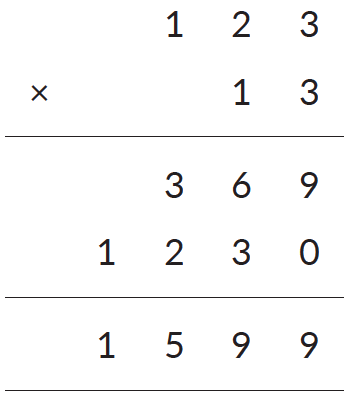The given example shows multiplication of of a 3-digit number $$(123)$$  and a 2-digit number $$(13)$$ using the standard algorithm. $$123$$ is the multiplicand, $$13$$ is the multiplies it can also be written as $$10 + 3.$$ To begin with $$3$$ is multiplied by $$123$$ and then $$10$$ is multiplied by $$123$$ resulting into product $$1599.$$

## How do you teach Multiplication and Division?

1. Use everyday objects to help children demonstrate multiplication.

2. Use Math manipulative such as colour counters to multiply quantities.

3. Get a laminated sheet with multiplication facts written on it. You can ask children to represent the fact using some stickers or bindis.

4. Put charts at home. Every once in a while question the child related to something given on the chart.

## Tips and Tricks

• In multiplication, the order of numbers does not matter (called the commutative property). So choose the order that you are more comfortable with. When relying on memory of multiplication tables, compared to $$9 \times 4,$$ students may remember $$4 \times 9$$ more easily.
• When multiplying three numbers, choose the two that can be multiplied easily.
E.g. $$5 \times 17 \times 2$$ will be difficult if we try to multiply $$5 \times 17$$ first. Instead, multiplying $$5$$ and $$2$$ gives $$10$$ which is easily multiplied by $$17$$ to get $$170.$$
• When multiplying a 2-digit number with a one-digit number, it sometimes helps to break the two-digit number as per the place values. Then multiply each part and add
E.g $$37 \times 4$$ can be solved mentally by breaking $$37$$ as $$30 + 7.$$
Then $$30 \times 4 = 120$$ and $$7 \times 4 = 28.$$ So the final answer is $$120 + 28 = 148.$$
While this may seem more tedious when written down, it is much easier to do mentally.
• Even if you don’t remember the multiplication fact, it can be easily mentally figured out. E.g. $$17 \times 9$$ is difficult to remember. But restructure this mentally as $$17 \times (10 - 1).$$ So the answer will be $$170 - 17 = 153.$$

### Common mistakes or misconceptions

• When multiplying by $$2$$ or more digits, children may miss adding a zero when multiplying by the digit in the tens place. This happens if they have not truly understood the reason for placing that zero or if they are in a hurry. See if they repeat this mistake with other similar problems. If yes, then it’s probably a conceptual error. Else it’s a silly mistake.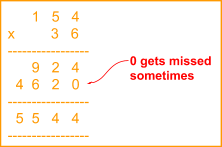• Children start by learning multiplication of whole number. This leads them to build a view that when two numbers are multiplied, the product will always be larger than the two numbers. This is not a problem as long as they are dealing with whole numbers but may become a stumbling block when fractions and decimals are introduced. This view does not hold when multiplying by quantities less than $$1.$$
• Guide your child through the concept of place value. One of the most common mistakes that arise while multiplying two numbers of 2-digit or greater is that children forget to transfer the place value when they move to the second number. That zero is there for a reason, it’s because you have moved one place higher. From the ones place to the tens place, which necessitates the addition of the zero. In essence you are just decomposing the lower number and adding the sub-parts. The concept is clearly shown in the example below:

Q1. Multiply the following numbers.

\begin{align} &a. \quad 15 \times 9 \\ &b. \quad 12 \times 13 \\ &c. \quad 132 \times 12 \\ &d. \quad 145 \times 20 \\ &e. \quad 112 \times 125 \end{align}

Introduction to Multiplication
Introduction to Multiplication
Multiplication
Multiplication
Multiplication